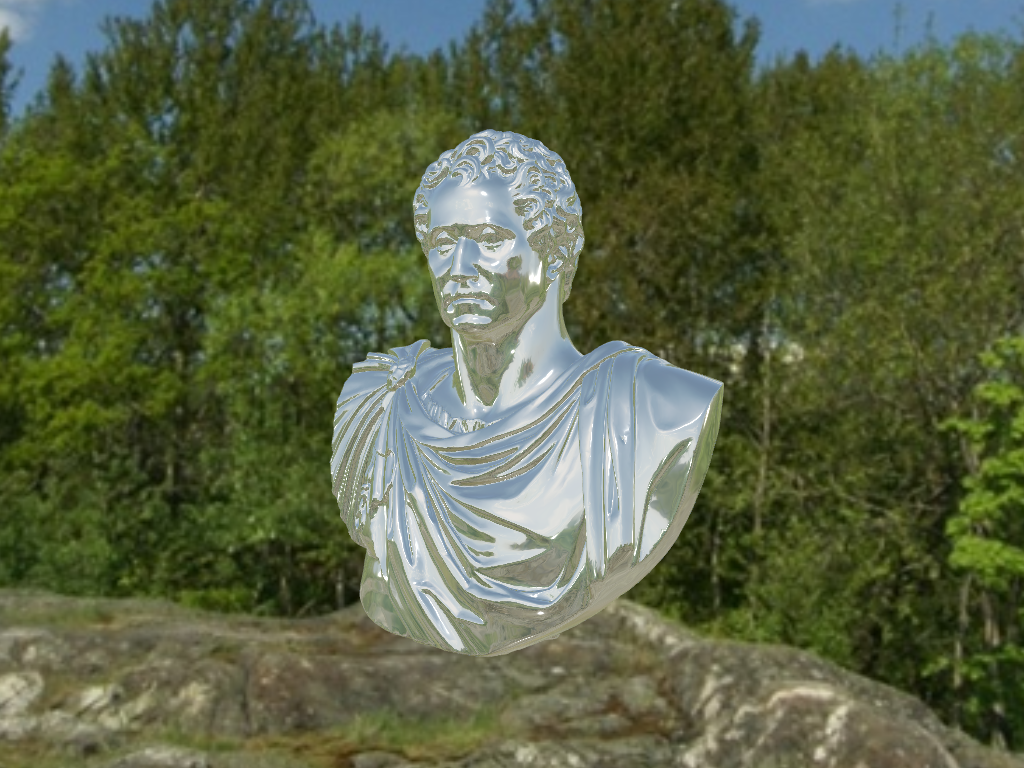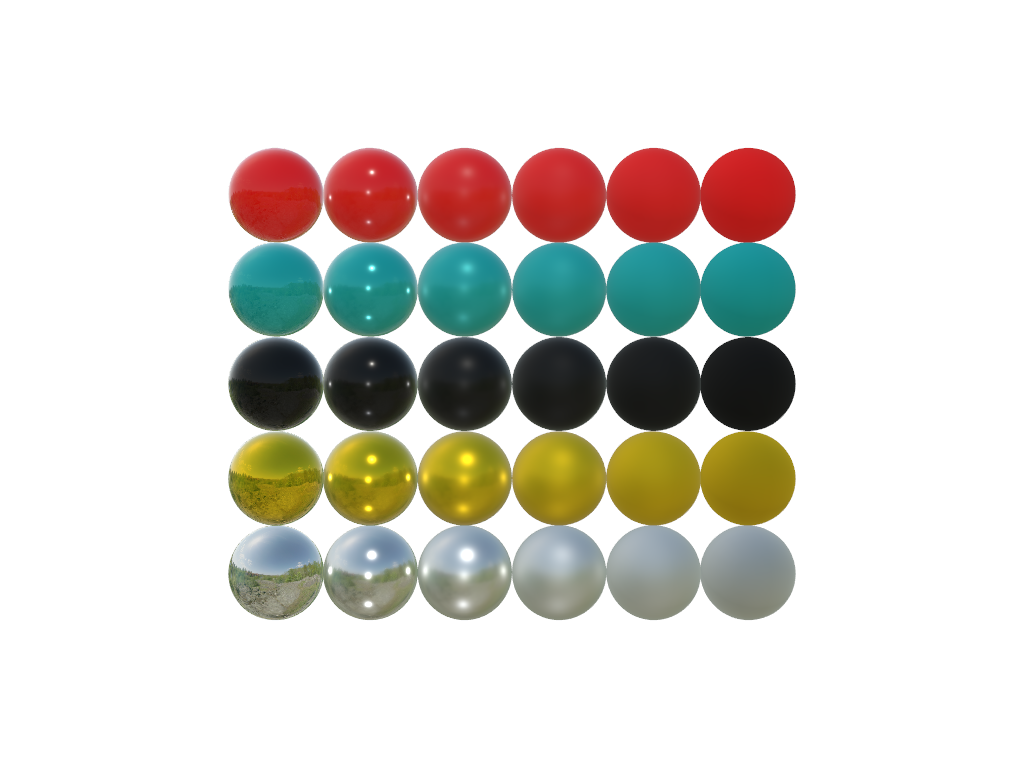# Physically Based Rendering#

VTK 9 introduced Physically Based Rendering (PBR) and we have exposed that functionality in PyVista. Read the blog about PBR for more details.

PBR is only supported for `pyvista.PolyData` and can be triggered via the `pbr` keyword argument of `add_mesh`. Also use the `metallic` and `roughness` arguments for further control.

Let’s show off this functionality by rendering a high quality mesh of a statue as though it were metallic.

```import pyvista as pv
from pyvista import examples

mesh.rotate_x(-90.0, inplace=True)  # rotate to orient with the skybox

```

Let’s render the mesh with a base color of “linen” to give it a metal looking finish.

```p = pv.Plotter()
p.set_environment_texture(cubemap)  # For reflecting the environment off the mesh
p.add_mesh(mesh, color='linen', pbr=True, metallic=0.8, roughness=0.1, diffuse=1)

# Define a nice camera perspective
cpos = [(-313.40, 66.09, 1000.61), (0.0, 0.0, 0.0), (0.018, 0.99, -0.06)]

p.show(cpos=cpos)
```Show the variation of the metallic and roughness parameters.

Plot with metallic increasing from left to right and roughness increasing from bottom to top.

```colors = ['red', 'teal', 'black', 'orange', 'silver']

p = pv.Plotter()
p.set_environment_texture(cubemap)

for i in range(5):
for j in range(6):
sphere = pv.Sphere(radius=0.5, center=(0.0, 4 - i, j))
p.add_mesh(sphere, color=colors[i], pbr=True, metallic=i / 4, roughness=j / 5)

p.view_vector((-1, 0, 0), (0, 1, 0))
p.show()
```Combine custom lighting and physically based rendering.

```# download louis model
mesh.rotate_z(140, inplace=True)

plotter = pv.Plotter(lighting=None)
plotter.set_background('black')
plotter.add_mesh(mesh, color='linen', pbr=True, metallic=0.5, roughness=0.5, diffuse=1)

# set up lighting
light = pv.Light((-2, 2, 0), (0, 0, 0), 'white')

light = pv.Light((2, 0, 0), (0, 0, 0), (0.7, 0.0862, 0.0549))

light = pv.Light((0, 0, 10), (0, 0, 0), 'white')